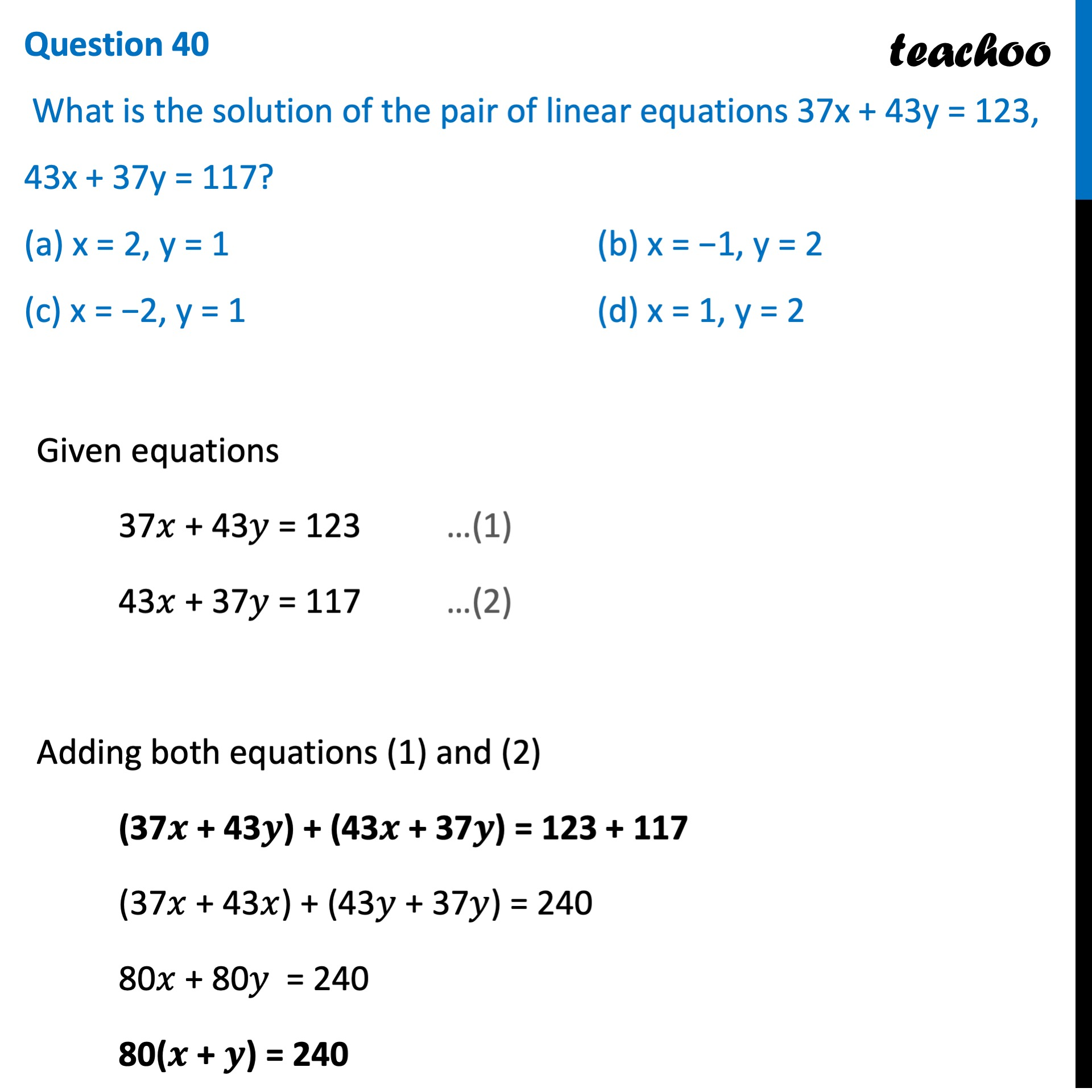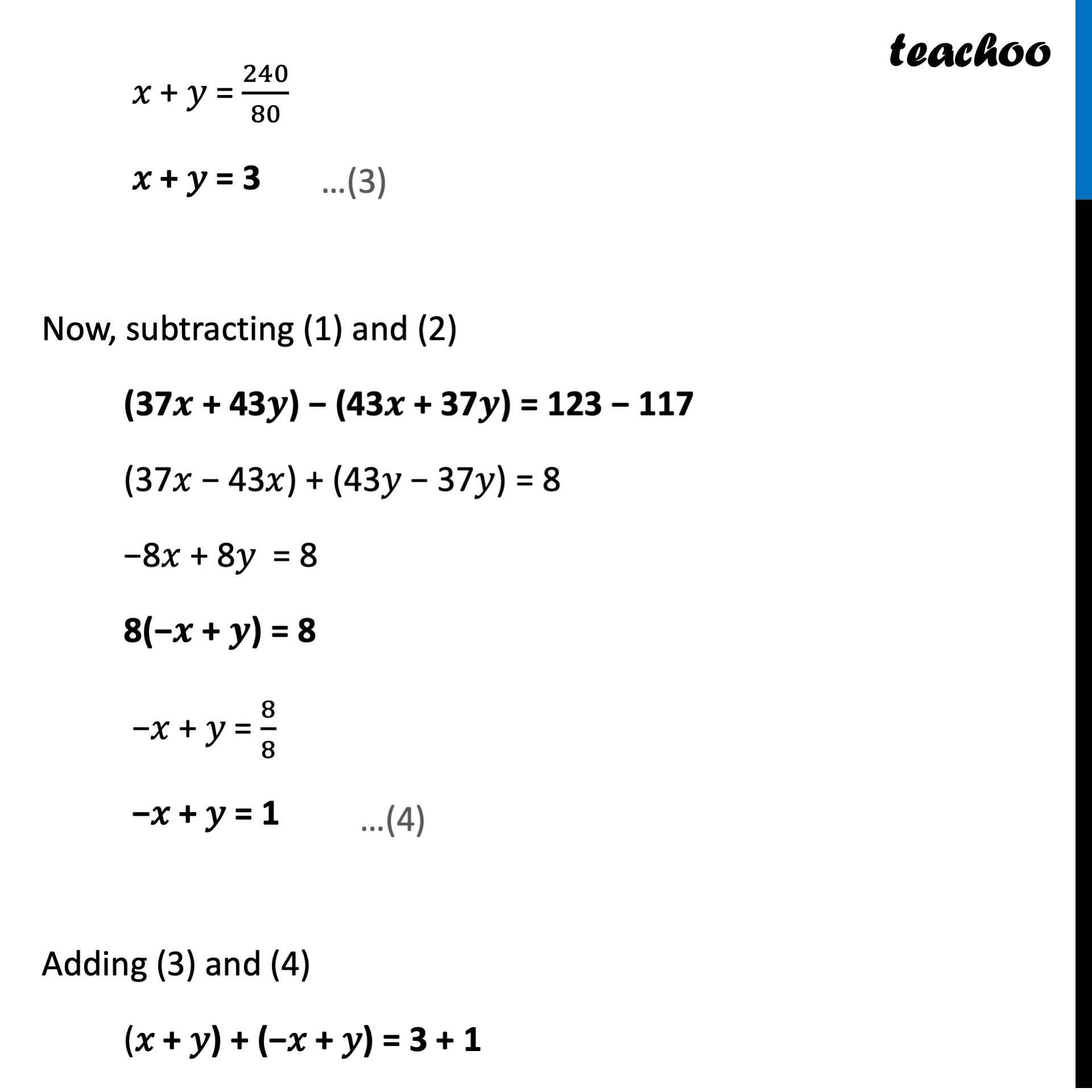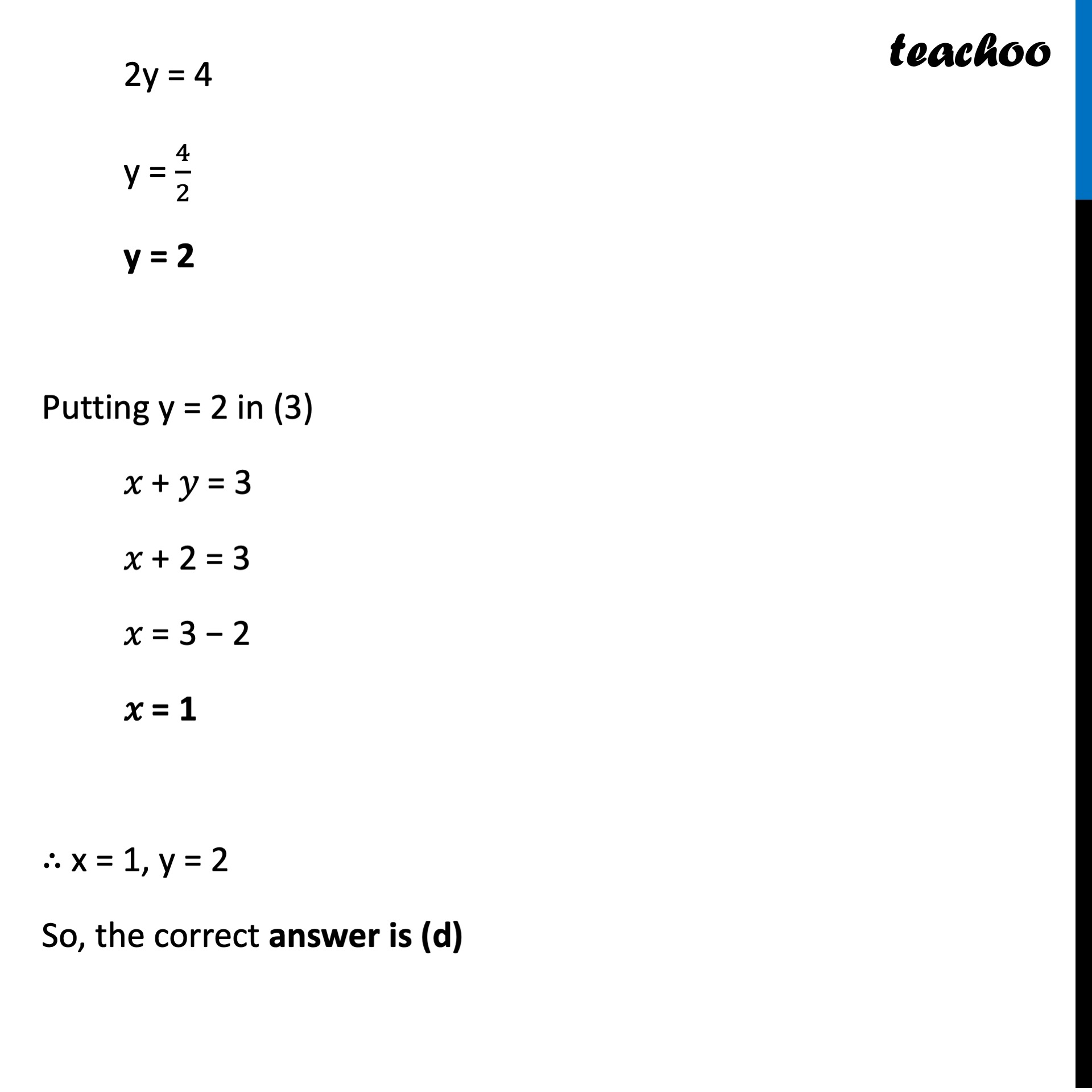CBSE Class 10 Sample Paper for 2022 Boards - Maths Basic [MCQ]

Class 10
Solutions of Sample Papers for Class 10 Boards

## (c) x = −2, y = 1   (d) x = 1, y = 2Learn in your speed, with individual attention - Teachoo Maths 1-on-1 Class

### Transcript

Question 40 What is the solution of the pair of linear equations 37x + 43y = 123, 43x + 37y = 117? (a) x = 2, y = 1 (b) x = −1, y = 2 (c) x = −2, y = 1 (d) x = 1, y = 2 Given equations 37𝑥 + 43𝑦 = 123 …(1) 43𝑥 + 37𝑦 = 117 …(2) Adding both equations (1) and (2) (37𝒙 + 43𝒚) + (43𝒙 + 37𝒚) = 123 + 117 (37𝑥 + 43𝑥) + (43𝑦 + 37𝑦) = 240 80𝑥 + 80𝑦 = 240 80(𝒙 + 𝒚) = 240 𝑥 + 𝑦 = 240/80 𝑥 + 𝑦 = 3 Now, subtracting (1) and (2) (37𝒙 + 43𝒚) − (43𝒙 + 37𝒚) = 123 − 117 (37𝑥 − 43𝑥) + (43𝑦 − 37𝑦) = 8 −8𝑥 + 8𝑦 = 8 8(−𝒙 + 𝒚) = 8 −𝑥 + 𝑦 = 8/8 −𝑥 + 𝑦 = 1 Adding (3) and (4) (𝑥 + 𝑦) + (−𝑥 + 𝑦) = 3 + 1 2y = 4 y = 4/2 y = 2 Putting y = 2 in (3) 𝑥 + 𝑦 = 3 𝑥 + 2 = 3 𝑥 = 3 − 2 𝑥 = 1 ∴ x = 1, y = 2 So, the correct answer is (d)